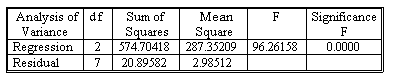### Seeing is believing!

Before you order, simply sign up for a free user account and in seconds you'll be experiencing the best in CFA exam preparation.

##### Subject 8. Is R2 Related to Statistical Significance?
Just as in the simple linear regression model, we can decompose the total variation in the dependent variation into two components: explained variation and unexplained variation.

R2 = (Total variation - Unexplained variation)/Total variation

Recall that R2 = 1 - SSE/SST, where SST = ∑(yt - y-bar)2, and SSE = ∑(e-hatti)2. The SST remains constant when another explanatory variable is added to a model because such an addition has no effect on the sum ∑(yt - y-bar)2. On the other hand, adding an additional explanatory variable to the model causes SSE to decline provided the estimated coefficient of the new variable is not exactly 0 and thus causes the value of R2 to increase. To this extent, then, the value of R2 depends on the number of explanatory variables included in the model. This causes a problem when we try to compare the goodness of fit of two models that have the same dependent variable but different number of explanatory variables.

Another measure of goodness-of-fit takes into account the number of explanatory variables included in an equation. The measure, called the adjusted R square and denoted R-bar2, is calculated as shown below:

Adjusted R2 = 1 - [SSE/(n - k - 1)]/[SST/(n - 1)]
= 1 - [(n-1)/(n - k - 1)] (1 - R2)

• R2 is always ≥ adjusted R2.
• When a new independent variable is added, adjusted R2 can decrease if adding that variable has only a small effect on R2.
• In fact, adjusted R2 can actually be negative if the correlation between the dependent variable and the independent variables is sufficient low.

Continue with our example discussed earlier:R2 = 1 - SSE/SST = 1 - 20.8958/(574.7042 + 20.8958) = 0.9649. Adjusted R2 = 1 - [(10 - 1)/(10 - 3)] (1 - 0.9649) = 0.9549. In fact, R2 and adjusted R2 are often presented in an ANOVA table.

Learning Outcome Statements

h. contrast and interpret the R2 and adjusted R2 in multiple regression;

i. evaluate how well a regression model explains the dependent variable by analyzing the output of the regression equation and an ANOVA table;

CFA® 2023 Level I Curriculum, Volume 1, Module 2

User Comment
but k is the number of all variables (including dependant and independant), or it is the number of independant variables + 1.
JimM I just googled adjusted R2 and most sites gave (n-k-1) in the formula.
arudkov 2 danlan - k - is the number of indep variables. +1 means interciept
In L2 Vol1, its n-k-1, not n-k.
Guess they corrected it. or maybe AnalystNotes is putting down the book for those who haven't read it, hehe. [i haven't, had to check, but Cmon guys, don't say bad things about CFA (textbook)]